# Planting and Growing Trees -Dynamically Adding Nodes Along with Data to an Existing Base Tree

Planting a tree (this is my terminology) is the process of creating a tree item in the Forms designer. There is no way to create a tree item dynamically. Initially it has to be created in the designer. A tree always has to be based on a query.

Growing a tree is the process of

• adding tree data sets under a given node (hierarchical sub-trees along with data under a given node).
• adding tree data elements (single nodes along with data).

Adding a data set amounts to dynamically adding child trees to an existing tree using a record group or query text. The base tree is and should be created at design time.

A child tree can be added either as a child of a specified node or below a specified node, both at a given offset. This is specified by the constants FTREE.PARENT_OFFSET or FTREE.SIBLING_OFFSET.

When added as a child of a specified node, the resulting node is at a level one below the specified node. The offset specifies in which node position, starting from the first child node, the new node can be added. It can be either (1- n ), where n is the number of child nodes under the specified node's parent, or the constant FTREE.LAST_CHILD. FTREE.LAST_CHILD specifies that the new node be added after the last node at the current level.

When added below a specified node ”that is, sibling ”the resulting root node is at the same level as the specified node. In this case, the offset is either FTREE.NEXT_NODE or FTREE.PREVIOUS_NODE.

To grow a tree, follow these steps:

1. Use FTREE.ADD_DATA_TREE and pass either a record group or query as the value for the data parameter:

```FTREE.ADD_TREE_DATA(item_id, node, offset_type, offset, data_source, data);
```
2. Specify either FTREE.PARENT_OFFSET (adding a child to the given node) or FTREE.SIBLING_OFFSET (sibling to the given node).
3. Specify the offset and corresponding start (base) node.
4. The most important item is the data source ”that is, RECORD_GROUP or QUERY_TEXT. This has to be a hierarchical query.

Again consider our example tree based on the EMP table in Figure 9.2.

We add the following two rows to the EMP table:

```insert into emp values (1111, 'DAVID', 'MANAGER', null,

'25-FEB-84', 8000, null, 10);

insert into emp values (2222, 'ROSE', 'ANALYST', 1111,

'25-FEB-85', 5000, null, 10);
```

We add the sub-tree returned by the following query as the last child of the node the user selects by a single-click :

```SELECT -1, LEVEL, ENAME, NULL, TO_CHAR(empno)

FROM emp

CONNECT BY PRIOR empno = mgr;
```

This is done when the user selects a node and then clicks a button labeled Add Sub-Tree. The following piece of code in the corresponding WHEN-BUTTON-PRESSED trigger does the job:

```
WHEN-BUTTON-PRESSED

DECLARE

item_id ITEM;

rg_id RECORDGROUP;

retcd NUMBER;

query_string VARCHAR2(1000);

BEGIN

item_id := FIND_ITEM('TREE_BLOCK.HTREE');

IF ID_NULl(item_id) THEN

MESSAGE('ERR: Invalid tree Item');

RAISE FORM_TRIGGER_FAILURE;

END IF;

query_string :=

'SELECT -1, LEVEL, ENAME, NULL, TO_CHAR(empno) FROM emp START WITH ename =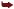''DAVID''CONNECT BY PRIOR empno = mgr';

IF NOT ID_NULl(rg_id) THEN

DELETE_GROUP(rg_id);

END IF;

retcd := POPULATE_GROUP(rg_id);

IF (retcd <> 0) THEN

MESSAGE('ERR: Tree Data Source Creation Failed');

RAISE FORM_TRIGGER_FAILURE;

END IF;FTREE.RECORD_GROUP, rg_id);

END;
```

The before and after tree changes are shown in Figures 9.3 and 9.4, respectively.

Figure 9.3. Tree structure before adding sub-tree. Mark the user-selected node labeled BLAKE.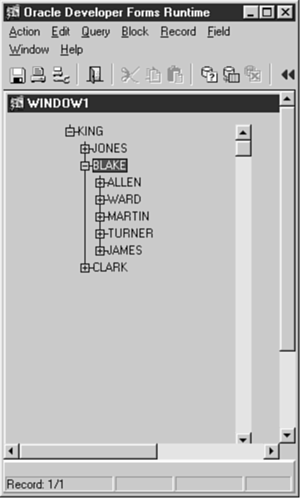Figure 9.4. Tree structure after adding sub-tree as the last child of the user-selected node labeled BLAKE.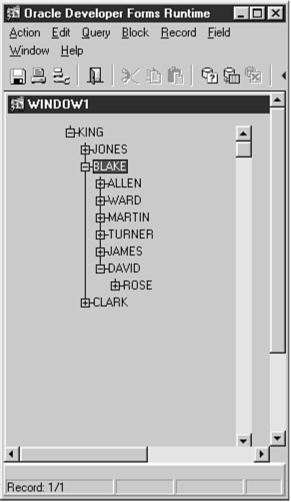Tip

No expanding or collapsing is done by FTREE.ADD_TREE_DATA.

Adding a Sub-tree to the Next Node of a Given Node and at the Same Level

So far, I have described how to add a sub-tree as a child node to a given node. Next I describe how to add a sibling of a given node. Adding a sibling adds a node next to a given node and at the same level. To demonstrate this, I will add a sibling to the very first node of the tree. To do this, the above trigger needs the following modification: Replace the constants FTREE.PARENT_OFFSET and FTREE.LAST_CHILD with FTREE.SIBLING_OFFSET and FTREE.NEXT_NODE as the values for offset_type and offset respectively.

The code with the given change is

```FTREE.RECORD_GROUP, rg_id);
```

The before and after tree changes are shown in Figures 9.5 and 9.6, respectively.

Figure 9.5. Tree structure before adding sub-tree. Mark the very first node labeled KING.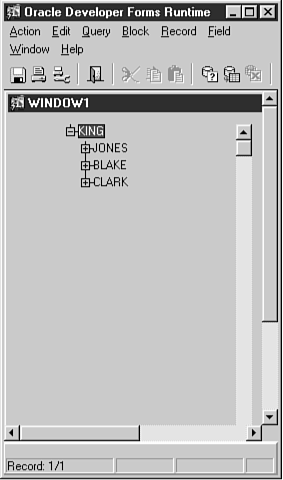Tip

A sibling node cannot be added by specifying the start node as FTREE.ROOT_NODE. Note that FTREE.ROOT_NODE is not the same as the very first top level node of the tree.

Figure 9.6. Tree structure after adding sub-tree as a sibling of the very first node labeled KING.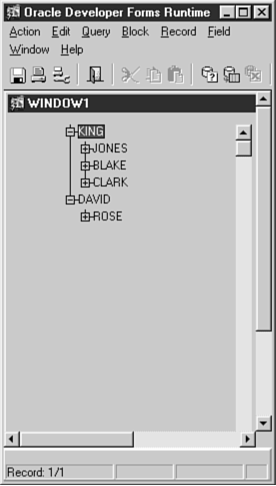Figure 9.6 shows the added sub-tree in a user-expanded fashion to illustrate the depth of query.

Adding a Sub-tree as the First Top-level Node of a Tree (Before the Node at Level 1)

This sub-section describes how to add a sub-tree to the very beginning of a tree. This means adding immediately after the root node. To do this, add a sibling to the very first node of the tree specifying FTREE.PREVIOUS_NODE as the offset. To do this, the above trigger line needs the following modification: Replace the offset value FTREE.NEXT_NODE with FTREE.PREVIOUS_NODE.

The code with the given change is

```FTREE.PREVIOUS_NODE, FTREE.RECORD_GROUP, rg_id);
```

The before and after tree changes are shown in Figures 9.5 and 9.7, respectively.

Figure 9.7. Tree structure after adding a sub-tree as the first top level node.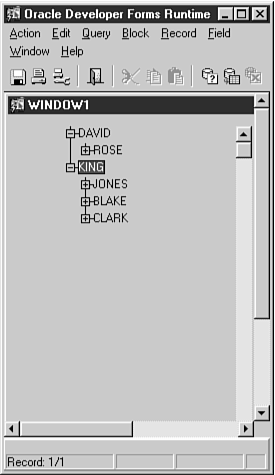Again, Figure 9.7 shows the added node in a user-expanded fashion to illustrate the depth of query.

Adding a data element means to dynamically add a single tree node to an existing tree using a value. The base tree is and should be created at design time.

A tree node can be added either as a child of a specified node or below a specified node, both at a given offset. This is specified by the constants FTREE.PARENT_OFFSET or FTREE.SIBLING_OFFSET.

When added as a child of a specified node, the resulting node is at a level one below the specified node. The offset specifies in which node position, starting from the first child node, the new node can be added. It can be either (1- n ) where n is the number of child nodes under the specified nodes parent, or the constant FTREE.LAST_CHILD. FTREE.LAST_CHILD specifies that the new node be added after the last node at the current level.

When added as below, a specified node ”that is, sibling ”the resulting root node is at the same level as the specified node. In this case, the offset is either FTREE.NEXT_NODE or FTREE.PREVIOUS_NODE.

1. Use FTREE.ADD_DATA_NODE and pass a value for the data parameter. Because the element to be added is a node, the state, label, icon, and value of the resulting node are also to be passed as parameters:

```FTREE.ADD_TREE_NODE(item_id, node, offset_type, offset, state, label, icon, value);
```
2. Specify either FTREE.PARENT_OFFSET (adding a child to the given node) or FTREE.SIBLING_OFFSET (sibling to the given node).
3. Specify the offset and corresponding start (base) node.
4. Most important is the quadruplet (state, label, icon, value). This has to be in conformity with normal tree values.

Consider our example tree based on EMP table. We will add a sub-node returned as the value of EMPNO of employee named SAMUEL, newly inserted as follows :

```insert into emp values (4444, 'SAMUEL', 'ANALYST', null, '26-FEB-94', 4000, NULL, 10);
```

This is done when the user selects a node and then clicks a special button labeled Add Sub-Node. The following piece of code in the corresponding WHEN-BUTTON-PRESSED trigger does the job:

```
WHEN-BUTTON-PRESSED

DECLARE

item_id ITEM;

v_empno NUMBER;

v_ename VARCHAR2(15);

BEGIN

BEGIN

SELECT empno, ename

INTO v_empno, v_ename

FROM emp

WHERE ename = SAMUEL;

EXCEPTION WHEN NO_DATA_FOUND THEN

RETURN;

END;

item_id := FIND_ITEM(TREE_BLOCK.HTREE);

IF ID_NULl(item_id) THEN

MESSAGE(ERR: Invalid tree Item);

RAISE FORM_TRIGGER_FAILURE;

END IF;FTREE.LAST_CHILD, FTREE.EXPANDED_NODE, v_ename,NULL,TO_CHAR(v_empno) );

END;
```

The before and after tree changes are shown in Figures 9.5 and 9.8, respectively.

Figure 9.8. Tree structure after adding a single node labeled SAMUEL as the last child of the user-selected node KING.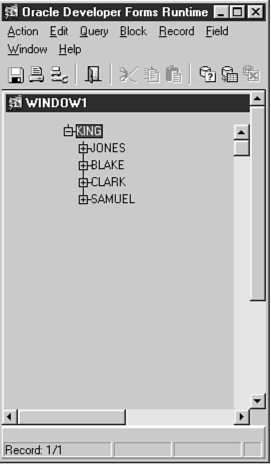Tip

No expanding or collapsing is done by FTREE.ADD_TREE_NODE.

Adding a Single Node as the Next Successive Node of a Given Node and at the Same Level

So far, I have described how to add a single child node to a given node. Next I describe how to add a single sibling of a given node. Adding a sibling adds a node beside and at the same level of a given node. To demonstrate this, I show how to add a single sibling to the very first node of the tree. To do this, the previous trigger needs the following modification: Replace the constants FTREE.PARENT_OFFSET and FTREE.LAST_CHILD with FTREE.SIBLING_OFFSET and FTREE.NEXT_NODE as the values for offset_type and offset, respectively. The code with the given change is:

```FTREE.EXPANDED_NODE, v_ename,NULL,TO_CHAR(v_empno) );
```

The before and after tree changes are shown in Figures 9.5 and 9.9, respectively.

Figure 9.9. Tree structure after adding a single node labeled SAMUEL as the sibling of the very first node labeled KING.Tip

Similarly to adding data sets, a sibling node cannot be added by specifying the start node as FTREE.ROOT_NODE. Note that FTREE.ROOT_NODE is not the same as the very first top level node of the tree.

Adding a Single Node as the First Top-level Node of a Tree (Before the First Node at Level 1)

This sub-section describes how to add a single node to the very beginning of a tree. This means adding immediately after the root node. To do this, add a single sibling to the very first node of the tree specifying FTREE.PREVIOUS_NODE as the offset. To do this the above trigger line needs the following modification: Replace the offset value FTREE.NEXT_NODE with FTREE.PREVIOUS_NODE.

The code with the given change is

```FTREE.PREVIOUS_NODE, FTREE.EXPANDED_NODE, v_ename,NULL,TO_CHAR(v_empno) );
```

The before and after tree changes are shown in Figures 9.5 and 9.10, respectively.

Figure 9.10. Tree structure after adding a single node as the very first top level node.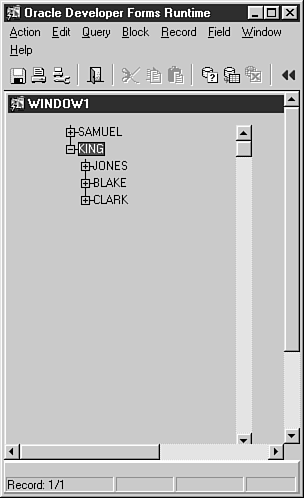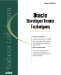Oracle Developer Forms Techniques
ISBN: 0672318466
EAN: 2147483647
Year: 2005
Pages: 115